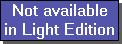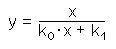The SDL Component Suite is an industry leading collection of components supporting scientific and engineering computing. Please visit the SDL Web site for more information....## CalcReciHyperbolFitUnit: SDL_math2 Class: Declaration: procedure CalcReciHyperbolFit (var k0,k1: double; var FitQual: double);

The procedure CalcReciHyperbolFit calculates the best fitting reciprocal hyperbola for a given set of data. The reciprocal hyperbola is determined by the equationThe values of x and y are given by the data samples, the parameters k0, and k1 are estimated by CalcReciHyperbolFit using a least squares approximation.

The data points [x,y] have to be entered using the routine EnterStatValue. A minimum number of 3 values is required in order to apply CalcReciHyperbolFit. Do not forget to reset the statistics calculation before entering any new data sets (use Init)

In addition to the parameters k0 and k1 CalcReciHyperbolFit returns the goodness of fit in the parameter FitQual. This quality of fit may vary between 0.0 and 1.0, indicating the best possible fit if FitQual equals 1.0.

 Hint 1: The quality of fit calculated by CalcReciHyperbolFit is not adjusted for the degree of freedoms in the regression parameters.

 Hint 2: Neither the x- nor the y-values are allowed to become than zero. The curve fitting algorithm raises an exception in the case of zero values.

 Example: This method is used in the following example program (see http://www.lohninger.com/examples.html for downloading the code): curvefit

Last Update: 2012-Oct-20